• PCA to reduce dimension data
• know how PCA works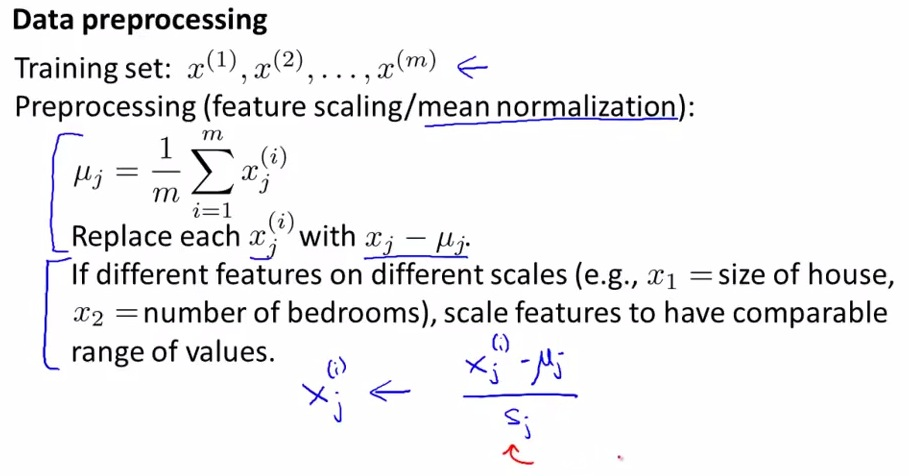• As we have above, is the training set of unsupervised learning that has unlabeled data, that is doesn't have y-value to label them
• We then do preprocessing by feature scaling / mean normalizatio, which is really similar of what we have in supervised learning
• We try to compute mean normalization that use input and mew to be equal to zero. This way we have reduce the data and minimalizing the error already.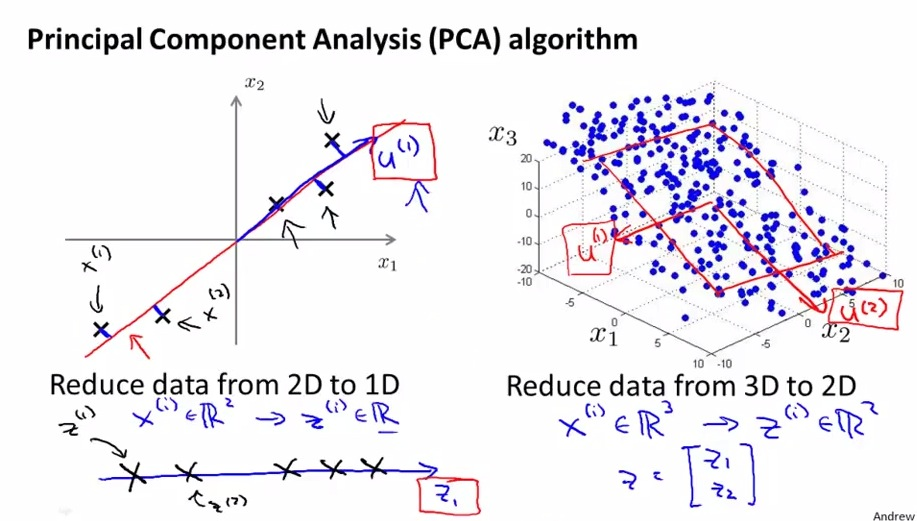• The graph above is the one we talked earlier, trying to reduce to lower dimension of the data
• Then we want to find a vector that has the same "eigenvector" of the line that we want to project(a.k.a same direction)
• We also have to take into account the position of the projection line. Example on the left, is we want to take 2D-position of the projection line
• Then we simply need single number 1-D position of the data in the projected line. Of course all the data projected not always in exact position of the line projected, so we have to keep in mind about the projection error for each data
• The graph in the right is trying to reduce the dimension of the data from 3D to 2D. the plane projected position, is also what we take as an input, which describe as Z value
• All the derrivation value is beyond the the scope of this class, but easily implemented for this particular problem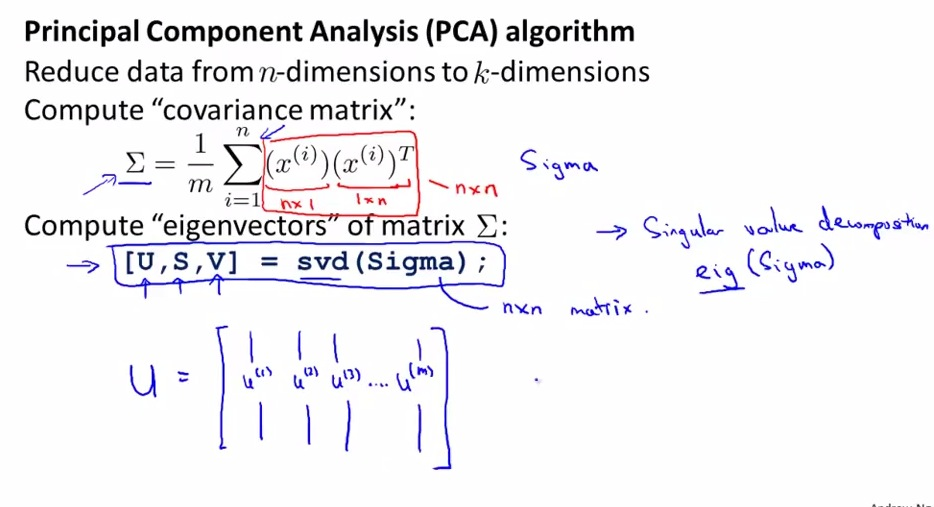• Ambigous symbol between sigma matrix and summation
• SVD is advanced linear algebra that implemented deeply in octave
• svd = eig  which svd more computationally more coherent
• different functions but this problem compute same thing
• there's always built in function to compute U S V
• As an input we have x matrix. We do the computation above (summation of X mult Xt to get Sigma matrix)
• Then from svd(Sigma), we extract Uk from U matrix)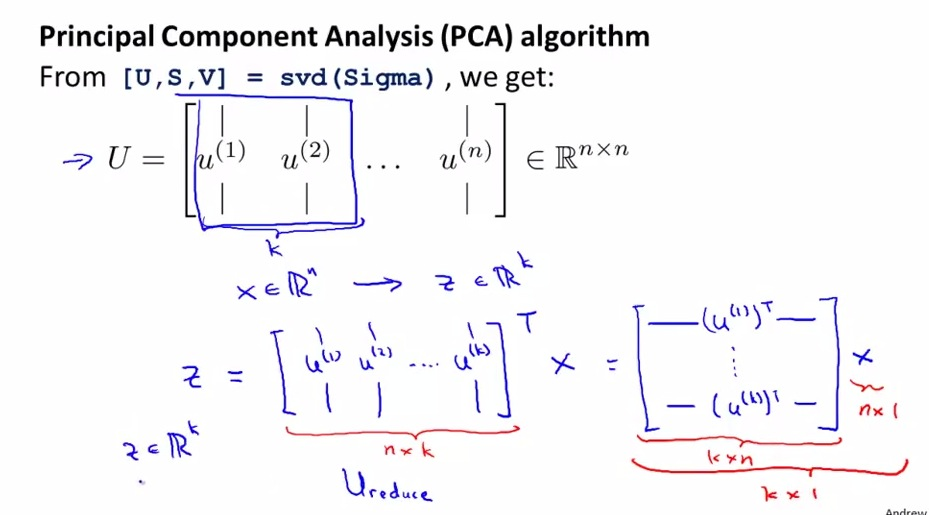• Ureduce that take U0 to Uk
• transpose  Ureduce  multiply x to produce Z vector, Z = Ut mult x
• x can be training set, test set, cv set
• put Ui for those three above if use set
• Don't forget that we fetch k units based on column of matrix U. so we fet U0-k column from U matrix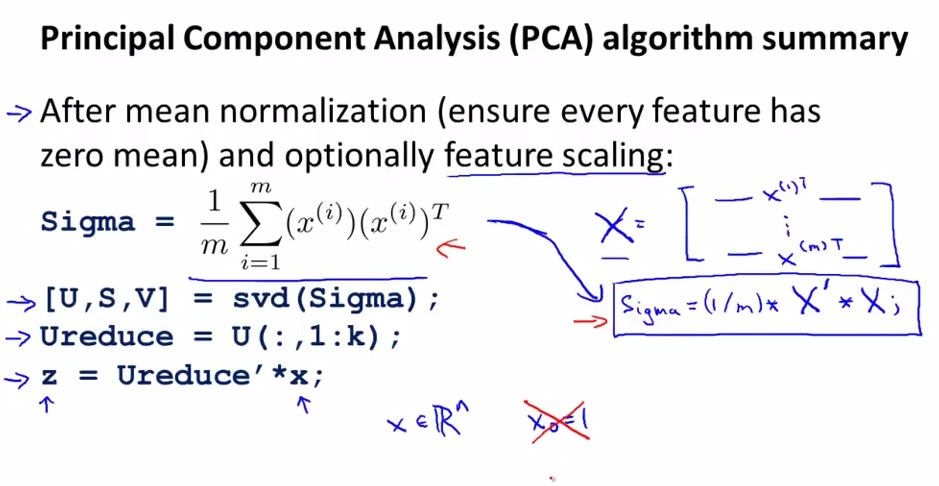• produce covariance sigma after preprocessing
• The blue writing is the vectorized implementation
• then use following step above to get z value
• Not using X0 convention
• Not give mathematival prove cause this beyon scope of this class
• Implemented this in octave/matlab give effective PCA Reduction algorithm
• In summary,first use pre-processing
• Then compute sigma based on the computation. And so on to get the value of Z
• The Z value that we get is the position of the projection of the lower dimension of the data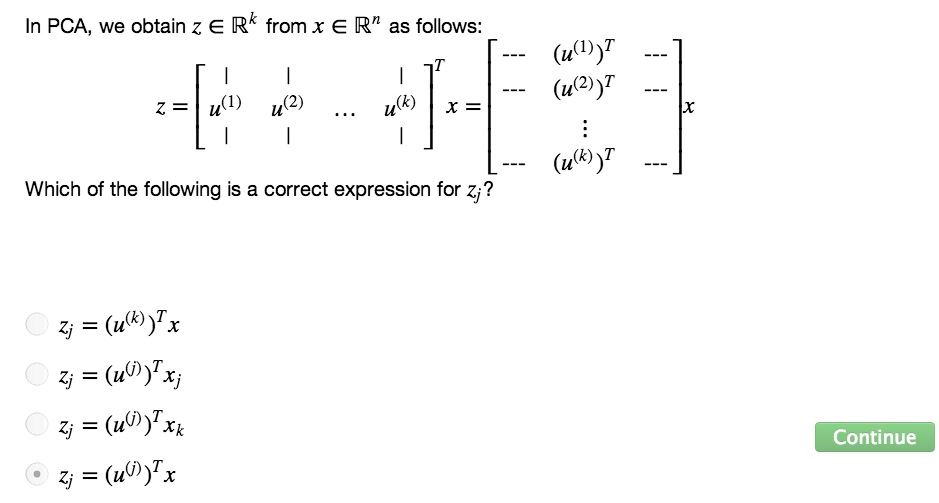• Mathematical prove of U,S,V to reduce the data dimension, really difficult beyond scope of this class. but in this particular problem, it should be implemented easily and it’s okay if we don’t understand it.
• minimizing squared projection error